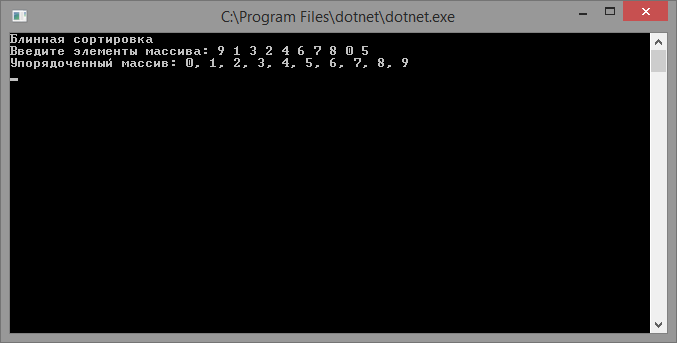# Pancake sort

Updated: 15.09.2019

Pancake sorting – an array sorting algorithm in which sorting is performed by flipping a part of the array.

## Description of pancake sorting algorithm

In this algorithm, only one operation is allowed to be applied to an array - a flip of a part of the array. And unlike other sorting methods, where they try to reduce the number of comparisons, this requires minimizing the number of flips.

The idea of ​​the algorithm is to move the maximum element to the end of the array for each pass. To do this, follow these steps:

1. At the beginning, select a subarray equal in length to the current array;
2. Find in it the position of the maximum element;
3. If the maximum element is not located at the end of the subarray, then:
• Turn the part of the array from the beginning to the maximum value;
• Flip the entire selected subarray;
4. Reduce the working area of ​​the array and go to the second step.

## Implementing pancake sorting

``````using System;

class program
{
// method to get the index of the maximum subarray element
static int IndexOfMax(int[] array, int n)
{
int result = 0;
for (var i = 1; i <= n; ++i)
{
if (array[i] > array[result])
{
result = i;
}
}

return result;
}

// method for flipping the array
static void Flip(int[] array, int end)
{
for (var start = 0; start < end; start++, end--)
{
var temp = array[start];
array[start] = array[end];
array[end] = temp;
}
}

// pancake sort
static int[] PancakeSort(int[] array)
{
for (var subArrayLength = array.Length - 1; subArrayLength > = 0; subArrayLength--)
{
// get the position of the maximum element of the subarray
var indexOfMax = IndexOfMax(array, subArrayLength);
if (indexOfMax != subArrayLength)
{
// flip the array to the maximum element index
// the maximum element of the subarray will be located at the beginning
Flip(array, indexOfMax);
// flip the entire subarray
Flip(array, subArrayLength);
}
}

return array;
}

static void Main(string[] args)
{
Console.WriteLine("Pancake sorting");
Console.Write("Enter elements of the array:");
var parts = Console.ReadLine().Split(new[] { "", ",", ";" }, StringSplitOptions.RemoveEmptyEntries);
var array = new int[parts.Length];
for (int i = 0; i < parts.Length; i++)
{
array[i] = Convert.ToInt32(parts[i]);
}

Console.WriteLine("Ordered array: {0}", string.Join(",", PancakeSort(array)));

The result of the program: